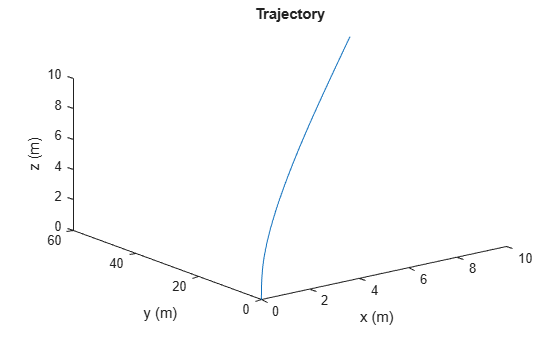# lookupPose

Obtain pose information for certain time

Since R2019b

## Syntax

``[position,orientation,velocity,acceleration,angularVelocity] = lookupPose(traj,sampleTimes)``

## Description

example

````[position,orientation,velocity,acceleration,angularVelocity] = lookupPose(traj,sampleTimes)` returns the pose information of the waypoint trajectory at the specified sample times. If any sample time is beyond the duration of the trajectory, the corresponding pose information is returned as `NaN`.```

## Examples

collapse all

Create a `waypointTrajectory` object that connects two waypoints. The velocity of the trajectory at the two waypoints is 0 $\mathrm{m}/\mathrm{s}$ and 10 $\mathrm{m}/\mathrm{s}$, respectively. Restrict the jerk limit to 0.5 $\mathrm{m}/{\mathrm{s}}^{3}$ to enable the trapezoidal acceleration profile.

```waypoints = [0 0 0; 10 50 10]; speeds = [0 10]; jerkLimit = 0.5; trajectory = waypointTrajectory(waypoints,GroundSpeed=speeds,JerkLimit=jerkLimit);```

Obtain the initial time and final time of the trajectory by querying the `TimeOfArrival` property. Create time stamps to sample the trajectory.

```t0 = trajectory.TimeOfArrival(1); tf = trajectory.TimeOfArrival(end); sampleTimes = linspace(t0,tf,100);```

Obtain the position, velocity, and acceleration information at these sampled time stamps using the `lookupPose` object function.

`[position,~,velocity,acceleration,~] = lookupPose(trajectory,sampleTimes);`

Plot the trajectory.

```figure() plot3(position(:,1),position(:,2),position(:,3)) xlabel("x (m)") ylabel("y (m)") zlabel("z (m)") title("Trajectory")```Plot the velocity profile.

```figure() subplot(3,1,1) plot(sampleTimes,velocity(:,1)); ylabel("v_x (m/s)") title("Velocity Profile") subplot(3,1,2) plot(sampleTimes,velocity(:,2)); ylabel("v_y (m/s)") subplot(3,1,3) plot(sampleTimes,velocity(:,3)); ylabel("v_z (m/s)") xlabel("Time (sec)")```Plot the acceleration profile. From the results, the acceleration profile of the planar motion is trapezoidal.

```figure() subplot(3,1,1) plot(sampleTimes,acceleration(:,1)); axis padded ylabel("a_x (m/s^2)") title("Acceleration Profile") subplot(3,1,2) plot(sampleTimes,acceleration(:,2)); ylabel("a_y (m/s^2)") axis padded subplot(3,1,3) plot(sampleTimes,acceleration(:,3)); ylabel("a_z (m/s^2)") xlabel("Time (sec)")```## Input Arguments

collapse all

Waypoint trajectory, specified as a `waypointTrajectory` object.

Sample times in seconds, specified as an M-element vector of nonnegative scalars.

## Output Arguments

collapse all

Position in the local navigation coordinate system in meters, returned as an M-by-3 matrix.

M is specified by the `sampleTimes` input.

Data Types: `double`

Orientation in the local navigation coordinate system, returned as an M-by-1 `quaternion` column vector or a 3-by-3-by-M real array.

Each quaternion or 3-by-3 rotation matrix is a frame rotation from the local navigation coordinate system to the current body coordinate system.

M is specified by the `sampleTimes` input.

Data Types: `double`

Velocity in the local navigation coordinate system in meters per second, returned as an M-by-3 matrix.

M is specified by the `sampleTimes` input.

Data Types: `double`

Acceleration in the local navigation coordinate system in meters per second squared, returned as an M-by-3 matrix.

M is specified by the `sampleTimes` input.

Data Types: `double`

Angular velocity in the local navigation coordinate system in radians per second, returned as an M-by-3 matrix.

M is specified by the `sampleTimes` input.

Data Types: `double`

## Version History

Introduced in R2019b